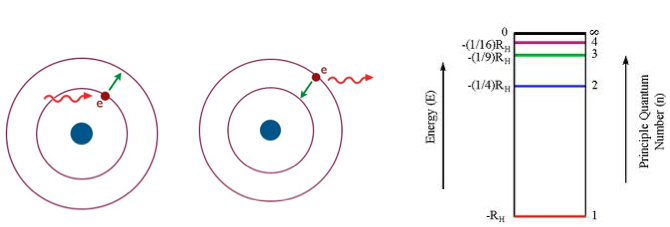# Problem: For a hydrogen atom, which case will result in an absorption spectrum with the highest frequency?a.  an electron transition from n = 1 → n = 2b.  an electron transitions from n = 1 → n = 4c.  an electron transition from n = 2 → n = 3d.  an electron transitions from n = 3 → n = 1e.  an electron transition from n = 4 → n = 3

###### FREE Expert Solution
93% (258 ratings)
###### Problem Details

For a hydrogen atom, which case will result in an absorption spectrum with the highest frequency?

a.  an electron transition from n = 1 → n = 2

b.  an electron transitions from n = 1 → n = 4

c.  an electron transition from n = 2 → n = 3

d.  an electron transitions from n = 3 → n = 1

e.  an electron transition from n = 4 → n = 3